# Non Verbal Reasoning - Figure Matrix

Exercise : Figure Matrix - Section 1
Directions to Solve

In each of the following questions, find out which of the answer figures (1), (2), (3) and (4) completes the figure matrix ?

6.

Select a suitable figure from the four alternatives that would complete the figure matrix.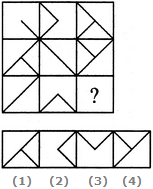1
2
3
4
Explanation:
The third figure in each row comprises of parts which are not common to the first two figures.

7.

Select a suitable figure from the four alternatives that would complete the figure matrix.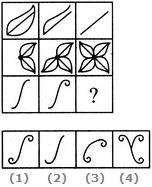1
2
3
4
Explanation:
The number of components in each row either increases or decreases from left to right. In the third row, it increases.

8.

Select a suitable figure from the four alternatives that would complete the figure matrix.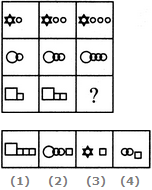1
2
3
4
Explanation:
In each row, the second figure is obtained from the first figure by increasing the number of smaller elements by one and the third figure is obtained from the second figure by increasing the number of smaller elements by one.

9.

Select a suitable figure from the four alternatives that would complete the figure matrix.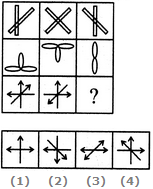1
2
3
4
Explanation:
The third figure in each row comprises of parts which are not common to the first two figures.

10.

Select a suitable figure from the four alternatives that would complete the figure matrix.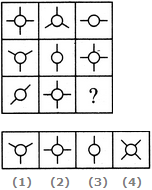1
2
3
4